## The circumference of a circle is 28. Contained in that circle is a smaller circle with area 36π. A point is selected at random from inside t

Question

The circumference of a circle is 28. Contained in that circle is a smaller circle with area 36π. A point is selected at random from inside the large circle. What is the probability the point also lies in the smaller circle?

in progress 0
6 months 2021-07-30T12:35:31+00:00 1 Answers 1 views 0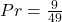Step-by-step explanation:

Given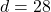— big circle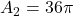— area of small circle

Required

Probability that a point selected lands on the small circle

Calculate the area of the big circle using;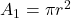Where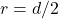So, we have: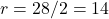This gives: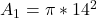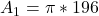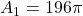The probability that a point selected lands on the small circle is calculated by dividing the area of the small cicle by the big circle

This gives: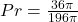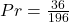Simplify